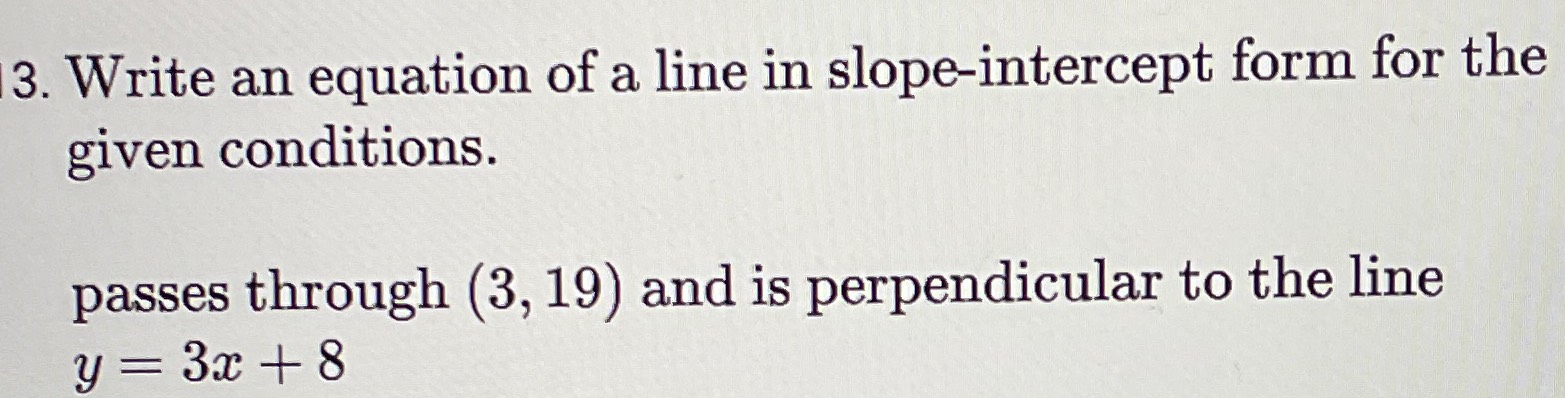### ¿Todavía tienes preguntas de matemáticas?

Pregunte a nuestros tutores expertos
Algebra
Pregunta3. Write an equation of a line in slope-intercept form for the given conditions.

passes through $$( 3,19 )$$ and is perpendicular to the line

$$y = 3 x + 8$$

$$y= -\frac{1}{3} x+ 20$$